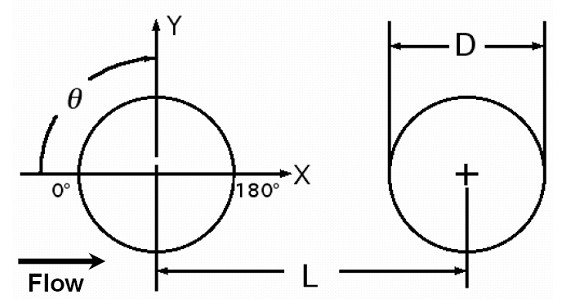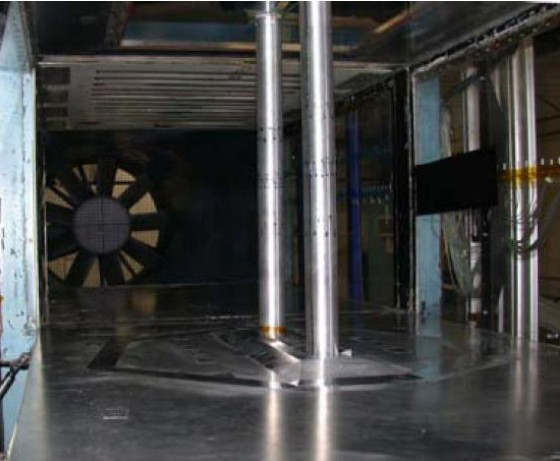# Test Case Study

## Brief Description of the Study Test Case

A detailed description of the chosen test case (TC with L = 3.7D) is available at this link. So here we present only its brief overview.

A schematic of the airflow past the TC configuration is shown in Figure 1. The model is comprised of two cylinders of equal diameter aligned with the streamwise flow direction. The polar angle, ${\theta }$, is measured from the upstream stagnation point and is positive in the clockwise direction.Figure 1: Schematic of TC configuration 

Geometric and regime parameters defining the test case are summarized in Table 1.

Table 1: Flow parameters
Parameter Notation Value
Reynolds number Re=$U_{0}D/\nu$1.66×105
Mach number M 0.128
Separation distance $L/D$3.7
TC aspect ratio $L_{z}/D$12.4
Cylinder diameter $D$0.05715 m
Free stream velocity $U_{0}$44 m/s
Free stream turbulence intensity $K$0.1%

The principal measured quantities by which the success or failure of CFD calculations are to be judged are as follows:

• Mean Flow
• Distributions of time-averaged pressure coefficient, $C_{p}\leq (p-p_{0})\geq (1/2\rho _{0}U_{0}^{2})$, over the surface of both cylinders;
• Distribution of time-averaged mean streamwise velocity ${\left.\langle u\rangle /U_{0}\right.}$along a line connecting the centres of the cylinders;
• Distributions of the root-mean-square (rms) of the pressure coefficient over the surface of both cylinders;
• Power spectral density of the pressure coefficient (dB/Hz versus Hz) on the upstream cylinder at $\theta$= 135°;
• Power spectral density of the pressure coefficient (dB/Hz versus Hz) on the downstream cylinder at $\theta$= 45°;
• Turbulence kinetic energy
• x – y cut of the field of time-averaged two-dimensional turbulent kinetic energy ${\text{TKE}}={\frac {1}{2}}\left(\langle u'u'\rangle +\langle \nu '\nu '\rangle \right)/U_{0}^{2}$;
• 2D TKE distribution along* y = 0;
• 2D TKE distribution along* x = 1.5 D (in the gap between the cylinders);
• 2D TKE distribution along* x = 4.45 D (0.75 D downstream of the centre of the rear cylinder).

All these and some other data are available on the web site of the BANC-I Workshop.

## Test Case Experiments

A detailed description of the experimental facility and measurement techniques is given in the original publications [2-4] and available on the web site of the BANC-I Workshop. So here we present only concise information about these aspects of the test case.Figure 2: TC configuration in the BART facility 

Experiments have been conducted in the Basic Aerodynamic Research Tunnel (BART) at NASA Langley Research Center (see Figure 2). This is a subsonic, atmospheric wind-tunnel for investigation of the fundamental characteristics of complex flow-fields. The tunnel has a closed test section with a height of 0.711 m, a width of 1.016 m, and a length of 3.048 m. The span size of the cylinders was equal to the entire BART tunnel height, thus resulting in the aspect ratio Lz / D = 12.4. The free stream velocity was set to 44 m/s giving a Reynolds number based on cylinder diameter equal to 1.66 × 105 and Mach number equal to 0.128 (flow temperature T = 292 K).

The free stream turbulence level was less than 0.10%. In the first series of the experiments [2, 3], in order to ensure a turbulent separation from the upstream cylinder at the considered Reynolds number, the boundary layers on this cylinder were tripped between azimuthal locations of 50 and 60 degrees from the leading stagnation point using a transition strip. For the downstream cylinder, it was assumed that trip-like effect of turbulent wake impingement from the upstream cylinder would automatically ensure turbulent separation. However, later on  it was found that the effect of tripping of the downstream cylinder at L/D = 3.7 is also rather tangible (resulted in reduced peaks in mean Cp distribution along the rear cylinder, accompanied by an earlier separation from the cylinder surface, a reduced pressure recovery, lower levels of mean TKE in the wake and reduced levels of peak surface pressure fluctuations). For this reason, exactly these (with tripping of both cylinders) experimental data  were used for the comparison with fully turbulent CFD.

In the course of experiments, steady and unsteady pressure measurements were carried out along with 2-D Particle Image Velocimetry (PIV) and hot-wire anemometry used for documenting the flow interaction around the two cylinders (mean streamlines and instantaneous vorticity fields, shedding frequencies and spectra).

Information on the data accuracy available in the original publications [2-4] is summarized in Table 2. Most absolute values are given based on nominal tunnel conditions or on an average data value. Percentage values are quoted for parameters where the uncertainty equations were posed in terms of the uncertainty relative to the nominal value of the parameter.

Table 2: Estimated Experimental Uncertainties
Quantity Uncertainty
Drag Coefficient 0.0005
PIV: Umean, Vmean 0.03 (normalized)
PIV: Spanwise velocity 1.8 (normalized)
PIV: TKE 4%
Power Spectral Density (PSD) 10 – 20%
Cp' rms 5 – 11%
Diameter, D; Sensor spacing Δz 0.005 inch

## CFD Methods

The key physical features of the UFR (Section 1) present significant difficulties for all the existing approaches to turbulence representation, whether from the standpoint of solution fidelity (for the conventional (U)RANS models) or in terms of computational expense for full LES (especially if the turbulent boundary layers are to be resolved). For this reason, most of the computational studies of multi-body flows, in general, and the TC configuration, in particular, are currently relying upon hybrid RANS-LES approaches. This is true also with regard to simulations carried out in the course of the BANC-I and II Workshops and in the framework of the ATAAC project, where different hybrid RANS-LES models of the DES type were used (see Table 3) .

Table 3: Summary of simulations
Partner Turbulence Modelling approach Compressible/Incompressible Lz Grid Side Walls
Beijing Tsinghua University BTU SST DDES Compressible 3D Mandatory Slip
German Aerospace Center, Göttingen DLR SA DDES Compressible 3D Mandatory Slip
New Technologies and Services, St.-Petersburg, Russia NTS SA DDES

SA IDDES

Incompressible and Compressible 3D, 16D Mandatory Slip
Technische Universität Berlin TUB SA DDES

SA IDDES

Incompressible 3D Mandatory Slip
SST - k - ω Shear Stress Transport model ; SA - Spalart-Allmaras model ; SA and SST DDES - Delayed DES based on the SA and SST models ; SA IDDES - Improved DDES based on the SA model 

1. Some participants of the BANC Workshops have used pure LES rather than DES-like approaches but these computations had the most difficulty simulating the high Reynolds aspects of the flow .

Contributed by: A. Garbaruk, M. Shur and M. Strelets — New Technologies and Services LLC (NTS) and St.-Petersburg State Polytechnic University